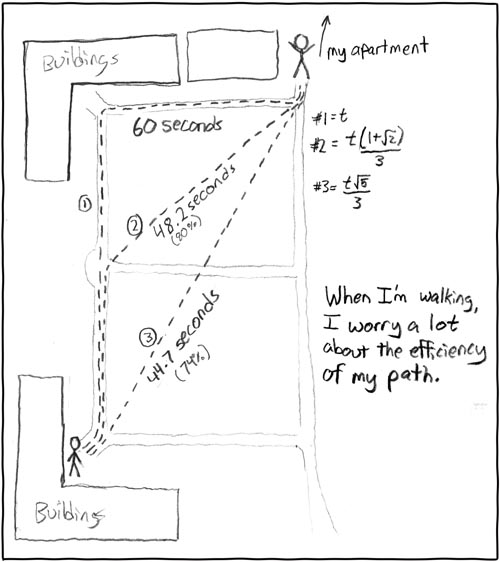# 85: Paths

 PathsTitle text: It's true, I think about this all the time.

## Explanation

This comic centers around the consideration of what is the shortest path available to a person traveling by foot. Cueball has to travel across a rectangular distance, which has an established path around the periphery. When Cueball follows these paths, he has to walk for 60 seconds. He realises that by ignoring the paths and taking the desire lines from corner to corner, his route will be shorter, and he calculates that he could cut up to 26% of his time. As a result, every time he has to travel this rectangle, he worries about the extra time taken as a result of following the path.

## Transcript

[Blueprint of a campus. Two buildings in the upper and lower left corners, respectively, and a rectangular lawn. A road encloses the lawn, another road traverses horizontally through the center of the lawn. The character is in the lower left and the upper right corner, where it says "my apartment".]
[Dashed line 1, from the lower-left along the road to the top-left corner, then to the top-right corner.] 60 seconds
[Dashed line 2, from the lower-left along the road up to the center crossroads, then diagonally over the lawn to the top-right corner.] 48 seconds (80%)
[Dashed line 3, diagonally from the lower-left to the top-right corner.] 44.7 seconds (74%)
My apartment
1. 1=t
2. 2=(t*(1+√2))/3
3. 3=(t*√5)/3
When I'm walking, I worry a lot about the efficiency of my path.add a comment! ⋅add a topic (use sparingly)! ⋅refresh comments!

# Discussion

This is the kind of thing that comes up in story problems in Calculus often. If you can travel in/over one medium at one speed, and in/over another medium at a different speed, what is the optimum path to minimize your travel time.
An example of this problem would be if there is a drowning swimmer 100 meters offshore, you are 300 meters from the point on the shoreline closest to the swimmer, and you can run at 15mph and swim at 2mph, how far do you run along the shoreline before going into the water to get to the swimmer as quickly as possible?
The fact that Randall shows two different paths over the "grass" makes me think that he was thinking more along the line of obsessively optimizing his path rather than about whether it might be acceptable or not to walk over the grass. -- mwburden 70.91.188.49 21:23, 13 December 2012 (UTC)

Along similar lines, this mathematician's dog uses Calculus (albeit at an intuitive, rather than mathematical level) to optimize the path that it takes to retrieve the ball from the water. -- mwburden 70.91.188.49 21:27, 13 December 2012 (UTC)

This particular situation is less interesting, since the walker's speed is the same for all three paths! This is seen by the times being directly proportional to the distances. Normally, the off-normal-path is at a lower speed, but some shorter path still gives the smallest time.DrMath 08:22, 14 October 2013 (UTC)

Where do the equations come from to figure out #2 & #3 - can anybody derive it? 108.162.219.185 (talk) (please sign your comments with ~~~~)

The equation #2 comes from the second route. t(1+√2)/3 is how far the second path takes the guy. If each block is a unit square, the diagonal to the corner is √2 while the next part is 1. The t/3 part is making it comparable to the first one (the first one is t despite it being 3 unit squares). Equation #3 is t√(5)/3. Plugging 1, 2, and √5 into wolfram|alpha for triangle side lengths makes it a right triange, so the √5 comes from the side length (assuming unit squares) while the t/3 makes it comparable to the first one.Mulan15262 (talk) 02:36, 1 December 2014 (UTC)

Interestingly enough, if the three sides are equal in time taken (20 seconds each), the time it would take for path #2 would be 20rt2 + 20, and path three would be roughly 40, which comes out to 60, 48.28, and 40 seconds by using very simple geometry. 108.162.237.179 (talk) (please sign your comments with ~~~~)

Based on his times, it is two squares with the same side length. Base on that geometry, Path #2 will be the hypotenuse of a 45 degree right triangle. t = √(20^2 + 20^2) = 20 * √2 = 48.28. Path #3 would be t = √(20^2 + 40^2) = 20 * √5 = 44.72. Not sure where you got roughly 40 from. Were you thinking of the sin of 30 degree rule where the hypotenuse is double the opposite side? In this case, the adjacent is double the opposite which puts the hypotenuse at √5 times the opposite.Flewk (talk) 17:10, 24 December 2015 (UTC)

Shouldn't this be added to Time management category? {{subst:Unsigned IP|172.68.62.4}}

What the hell, no unsigned templates here? Anyway, I'm wondering why there's any worry. Couldn't he just cut across the grass diagonally? Sidewalk-conformist. — Kazvorpal (talk) 22:39, 14 August 2019 (UTC)